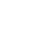Select Page# Numerical analyses / FEM calculations 2D/3D

Design calculation is possible for every subsoil…

• Groundwater flow calculations
• Stability verifications (PHI/c reduction)
• Deformation calculations
• 2D and 3D simulations
• Consolidation calculations
• Tunnel calculations
• Earthquake verifications / calculations in the time domain
• Recalculation of failure states
• Impact and collision analyses

## Numerical analyses / FEM calculations

Design calculation is possible for every subsoil…we have the right methods and tools to simulate and calculate your problem in 2D or in 3D. Besides Plaxis, we use OPTUM in 2D or 3D. Whether it is groundwater modelling, stability of complex systems or earthquake analyses in the time domain – we can find a solution. Whether with the FE method or the limit equilibrium method, we will be happy to determine the serviceability limit states (SLS) and the ultimate limit states (ULS) for your specific task.

## Numerical analyses / FEM calculations

Design calculation is possible for every subsoil…we have the right methods and tools to simulate and calculate your problem in 2D or in 3D. Besides Plaxis, we use OPTUM in 2D or 3D. Whether it is groundwater modelling, stability of complex systems or earthquake analyses in the time domain – we can find a solution. Whether with the FE method or the limit equilibrium method, we will be happy to determine the serviceability limit states (SLS) and the ultimate limit states (ULS) for your specific task.